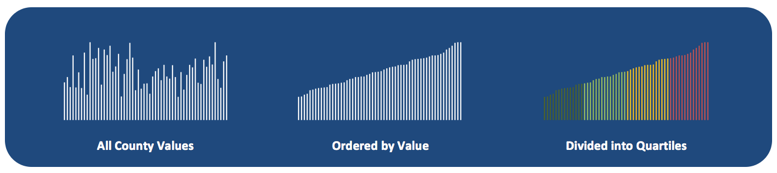# Indicator Measurement Glossary Terms

Prevalence: The total number of cases of a disease within a population at a given point in time. Prevalence is often given as a rate (the number of total cases divided by the number of individuals at risk for the disease). Prevalence measures how much of a disease is in the population and can be thought of as a snapshot of a disease's progression in the population.

Incidence: The number of new cases of a disease within a population. Incidence is often given as a rate (the number of new cases divided by the number of individuals at risk for the disease). Incidence measures the rate of occurrence of a disease in the population.

Rate: A measure or ratio of one quantity against another. Rates are often used when describing the presence of disease within a population.

Ratio: An explanation of relationship between two amounts that shows the number of times one value contains or is contained within the other.

Age-Adjusted: Age adjustment, also called age standardization, is a technique used to allow populations to be compared when the age profiles of the populations are quite different. For indicators, the default rate type is age-adjusted (as opposed to crude), when age-adjusted rates are provided by the source. Crude rates are used when age-adjusted rates are not available from the source.

Confidence Interval: The confidence interval describes how well a survey represents the population it refers to. Consider a survey that measured the obesity rate as 35% with a 95% confidence level and a margin of error of 5%. In this scenario, 95 out of 100 times the survey is conducted, the measured percentage of the population that is obese falls between 30% and 40% (the confidence interval).

Margin of Error: The margin of error is another term used to describe how well a survey represents the population it refers to. In the above example, the margin of error is 5%.

Median: The value at the midpoint of a distribution of values ordered from smallest to largest.

Distribution: A distribution is created by taking all zip code, county, or state values, ordering them from smallest to largest, and dividing them into quartiles based on their order.Unstable: A value that is marked unstable indicates a rate where small changes in the input will result in large changes in the rate. This is often due to small counts in the numerator or denominator (the input) used to calculate a rate. Unstable rates should be interpreted with caution because it is difficult to differentiate between random and true changes in the rates.

Jenks’ Natural Breaks: A way to classify data values into optimal groups, often used in epidemiological mapping. The natural breaks method reduces the variance within groups of values and maximizes variance between groups of values.